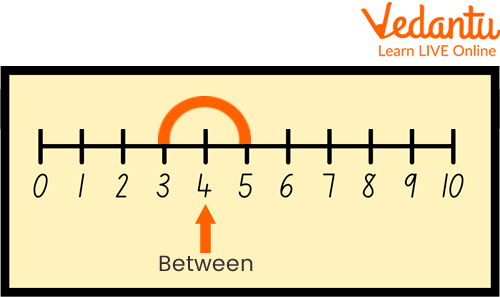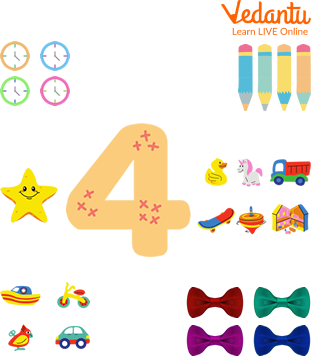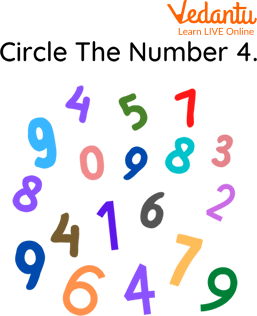Courses
Courses for Kids
Free study material
Offline Centres
More

# How to Write Number 4?Last updated date: 29th Nov 2023
Total views: 113.4k
Views today: 1.13k## An Overview of the Number 4

Are you stuck thinking about how to teach number 4 to your child? If yes, then you came across the perfect spot that will assist you to make your child learn how to write 4. Here, we have discussed the number 4, its different writing forms, its representation on the number line and how to write 4 in style. This article would not only help the parents but also will guide the children in learning how to write number 4. Now, let us proceed with this interesting topic here.

## What is the Number 4?

Number 4 can be defined as a number, numeral or digit which is the smallest composite natural number that comes after 3 and before 5. In many East Asian Countries, the number 4 is assumed to be unfortunate.

## Number 4 with Objects

Number 4 is the counting number. In the below-given picture, number 4 is shown by using the animals. The use of graphics helps the parents and teachers to make the children aware of how to write 4 easily.Showing the Number 4 with Objects

## How to Write Number 4?

One should often practise the number 4 by counting and writing it down to learn it quickly. Below are some workshops based on the "dotted line" phenomena that can aid kids in learning the number 4 easily.Showing the Way of Writing 4 on the Dotted Line

1. How to Write 4 in Roman Numerals?

The number 4 is written by placing I before V in roman numerals, i.e. lV. It denotes a number which is one less than 5.

2. How to write 4 in Style?

The number 4 can be written uniquely in a creative style using calligraphy. The given graphic denotes how to write 4 in style. Students need to repeatedly write it to understand it in a better way.

## Different Forms of Writing 4

The number 4 can be written in different forms such as:

• In Number Names

The number 4 is represented as four when written as a number name.

• In Ordinal Form

Number 4 is illustrated as fourth or 4th when written in an ordinal name.

• In Roman Numerals

When written as a roman numeral, the number 4 is represented by the letter lV.

• In Square Form

The number 4 is also expressed in terms of the square of a number, i.e., the number 4 is written as the square of the number 2, i.e., 2².

## Solved Examples

1. The number four lies between which two numbers?

Ans: As the number 4 is a counting number, therefore, it must lie between two numbers. Further, it is greater than the number 3 and less than the number 5. So, it lies between 3 and 5 whereas 3 is the predecessor of 4 while 5 is its successor. Thus, the number 4 lies between 3 and 5.Number 4

2. What happens when the number 4 is increased by 1?

Ans: When the natural number 1 gets added to the number 4, then 4 gets increased to the following number 5.

Thus, the number obtained after increasing 4 by 1 is the number 5.

## Practice Problems

Till now, the children would have gained a clear image of the number 4 in their mind which would help them to identify it. Some Number 4 Preschool Activities in the form of worksheets are given below that will help in evaluating how much the children have gained through the reading.

1. Count the objects and match with the number 4.Showing Number 4 Preschool Activities, based on the Concept of Count and MatchShowing Number 4 Preschool Activities, based on Circling the Number 4.

## Summary

Numbers serve as the building blocks of memorising Mathematics. These are useful not only in Mathematics but also in real life. This article covers every topic related to the number 4, including how to write the number four in roman numerals and number 4 preschool activities, by using several graphics so that children would easily grab the topic. The language used in this writing is kept simple and attractive to make the understanding of the concepts quite easy for the students. We hope you enjoyed reading it.

## FAQs on How to Write Number 4?

1. Explain writing by tracing technique.

Writing by tracing technique means tracing the already written numbers or letters. It helps the kids in learning how to write just by writing over the already written letters or numbers.

2. Is the use of flashcards effective in teaching numbers to the kids?

Yes, the use of flashcards helps the kids in learning the numbers quickly and easily by recognizing them.

3. What do you mean by the count and write phenomenon?

Count and write phenomenon states that firstly, one should count the number of given objects and then write the number obtained as result in the space provided aside. It serves as a useful tool in learning numbers easily.